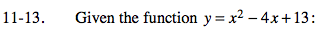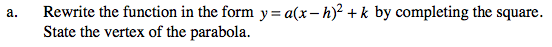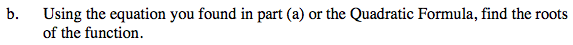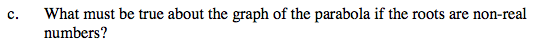Home > PC > Chapter 11 > Lesson 11.1.1 > Problem11-13

11-13.
1. Given the function y = x2 − 4x + 13: Homework Help ✎

1. Rewrite the function in the form y = a(x − h)2 + k by completing the square. State the vertex of the parabola.

2. Using the equation you found in part (a) or the Quadratic Formula, find the roots of the function.

3. What must be true about the graph of the parabola if the roots are non-real numbers?$\textit{y}-13+?=\textit{x}^2-4\textit{x}+? \: so \: that \: \textit{y}-\textit{k}=(\textit{x}-\textit{h})^2$The roots are the x-intercepts. Let y = 0 to find the x-intercepts.It has no x-intercepts.